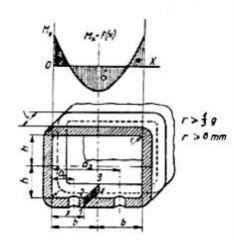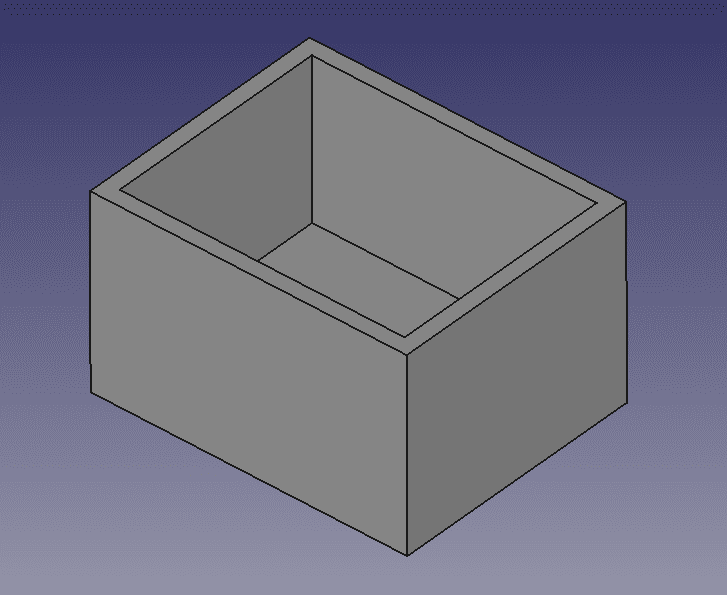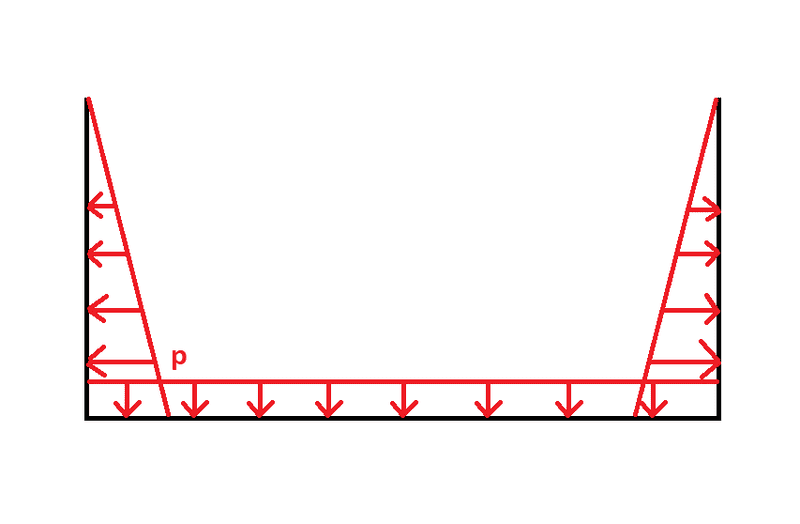# Stress in rectangular vessel subjected to hydrostatic pressure

• FEAnalyst

#### FEAnalyst

TL;DR Summary
How to calculate the maximum stress in a rectangular pressure vessel subjected to hydrostatic pressure?
Hi,

it's not easy to find formulas for stress in rectangular pressure vessels. However, I've found some in a Polish book titled "Podstawy konstrukcji aparatury chemicznej" (Fundamentals of the design of chemical process equipment) by J. Pikon. The problem is that the book provides equations for wall thickness and bending moment for the case of uniform pressure and only for wall thickness for the case of hydrostatic pressure. Here's the latter equation: $$g=0,5B \sqrt{\frac{p_{h}}{k}}$$ where: ##B## - width (length of a shorter side), ##p_{h}=\rho g H## - hydrostatic pressure, ##k## - allowable stress. I was thinking about converting this formula so that it solves for the stress: $$\sigma=\frac{p_{h}B^{2}}{4g^{2}}$$ but I'm not sure if this approach is correct. Maybe I should try to modify the equation for bending moment in a vessel subjected to uniform pressure instead. The question is how to do it though.

Pressurized vessels are seldom rectangular.
When they must have that shape, they normally have strong external reinforcing ribs that mainly prevent the welding of the edges to be bent by any deformation of the flat sides (keeping the L-shaped intersection at 90 degrees).
Those ribs reinforce the flat sections against the internal pressure, so resistance does not depend on the thickness of the material alone.

Pressurized vessels are seldom rectangular.
When they must have that shape, they normally have strong external reinforcing ribs that mainly prevent the welding of the edges to be bent by any deformation of the flat sides (keeping the L-shaped intersection at 90 degrees).
Those ribs reinforce the flat sections against the internal pressure, so resistance does not depend on the thickness of the material alone.
Yeah, I know. The book also covers ribs. For a case with hydrostatic pressure, they are treated like a beam with two supports and a triangular distributed load. However, my considerations are purely theoretical - I'm preparing a benchmark for finite element analysis software.

•Lnewqban
What is a rectangular pressure vessel. Can you please provide a diagram?

What is a rectangular pressure vessel. Can you please provide a diagram?
The aforementioned book provides only this diagram for the case of uniform pressure:But the structure may look like this (ignoring any ribs, fillets and holes):Of course, it's normally closed but for this analysis, it shouldn't really matter.

I should add that I've also found formulas for stress in this type of pressure vessel in the book titled “Structural Analysis and Design of Process Equipment” written by M.H. Jawad and J.R. Farr but equations there are only for the case of uniform pressure.

I should add that I've also found formulas for stress in this type of pressure vessel in the book titled “Structural Analysis and Design of Process Equipment” written by M.H. Jawad and J.R. Farr but equations there are only for the case of uniform pressure.

If you are talking about a gas or fluid, how would you get nonuniform pressure in a container?

Paging @jrmichler

If you are talking about a gas or fluid, how would you get nonuniform pressure in a container?
Hydrostatic pressure increases with depth.But it seems that I've found a solution to this problem. Books about reinforced concrete structures describe a specific approach to the calculation of rectangular pressure vessels - treating walls as individual plates. I've found proper equations for maximum stresses in "Roark's Formulas for Stress and Strain", assuming the case of a plate with three edges fixed and one edge free, subjected to triangular distributed load. The results are in very good agreement with FEA.

•Tom.G and Lnewqban